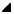# gmp_lib.mpz_kronecker_ui Method

#### GMP Native Interface for .NET

 gmp_libmpz_kronecker_ui Method
Calculate the Jacobi symbol (a/b) with the Kronecker extension (a/2) = (2/a) when a odd, or (a/2) = 0 when a even.

Namespace:  Math.Gmp.Native
Assembly:  Math.Gmp.Native (in Math.Gmp.Native.dll) Version: 1.0.0.0 (1.0.0.0)Syntax
```public static int mpz_kronecker_ui(
mpz_t a,
uint b
)```
```Public Shared Function mpz_kronecker_ui (
a As mpz_t,
b As UInteger
) As Integer```
```public:
static int mpz_kronecker_ui(
mpz_t^ a,
unsigned int b
)```
```static member mpz_kronecker_ui :
a : mpz_t *
b : uint32 -> int
```

#### Parameters

a
Type: Math.Gmp.Nativempz_t
The first operand integer.
b
Type: SystemUInt32
The second operand integer.

#### Return Value

Type: Int32
The Jacobi symbol (a/b) with the Kronecker extension (a/2) = (2/a) when a odd, or (a/2) = 0 when a even.Remarks

When b is odd the Jacobi symbol and Kronecker symbol are identical, so mpz_kronecker_ui, etc. can be used for mixed precision Jacobi symbols too.Examples
```// Create, initialize, and set the value of a to 15.
mpz_t a = new mpz_t();
gmp_lib.mpz_init_set_ui(a, 15U);

// Assert that the Kronecker symbol of (a/4) is 1.
Assert.IsTrue(gmp_lib.mpz_kronecker_ui(a, 4U) == 1);

// Release unmanaged memory allocated for a.
gmp_lib.mpz_clear(a);```
```' Create, initialize, and set the value of a to 15.
Dim a As New mpz_t()
gmp_lib.mpz_init_set_ui(a, 15UI)

' Assert that the Kronecker symbol of (a/4) is 1.
Assert.IsTrue(gmp_lib.mpz_kronecker_ui(a, 4UI) = 1)

' Release unmanaged memory allocated for a.
gmp_lib.mpz_clear(a)```

No code example is currently available or this language may not be supported.

No code example is currently available or this language may not be supported.See Also